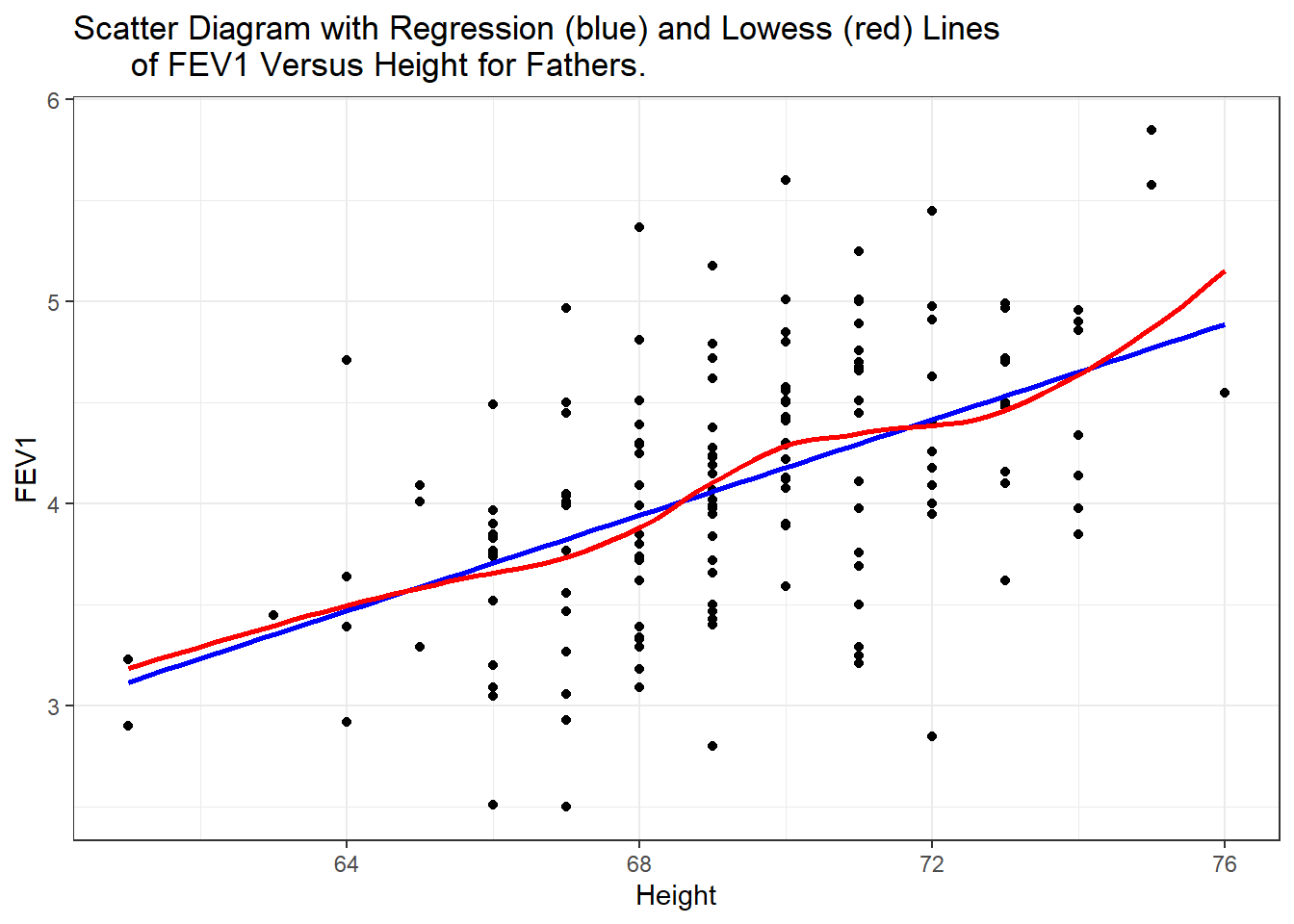## 7.5 Example

Returning to the Lung function data set from PMA6, lets analyze the relationship between height and FEV for fathers in this data set.

ggplot(fev, aes(y=FFEV1, x=FHEIGHT)) + geom_point() +
xlab("Height") + ylab("FEV1") +
ggtitle("Scatter Diagram with Regression (blue) and Lowess (red) Lines
of FEV1 Versus Height for Fathers.") +
geom_smooth(method="lm", se=FALSE, col="blue") +
geom_smooth(se=FALSE, col="red")There does appear to be a tendency for taller men to have higher FEV1. The trend is linear, the red lowess trend line follows the blue linear fit line quite well.

Let’s fit a linear model and report the regression parameter estimates.

fev.dad.model <- lm(FFEV1 ~ FHEIGHT, data=fev)
broom::tidy(fev.dad.model) |> kable(digits=3)
term estimate std.error statistic p.value
(Intercept) -4.087 1.152 -3.548 0.001
FHEIGHT 0.118 0.017 7.106 0.000

The least squares equation is $$Y = -4.09 + 0.118X$$. We can calculate the confidence interval for that estimate using the confint function.

confint(fev.dad.model) |> kable(digits=3)
2.5 % 97.5 %
(Intercept) -6.363 -1.810
FHEIGHT 0.085 0.151

For ever inch taller a father is, his FEV1 measurement significantly increases by .12 (95%CI: .09, .15, p<.0001).